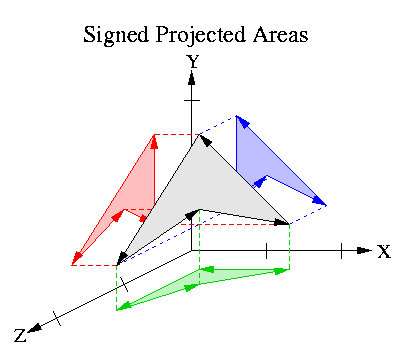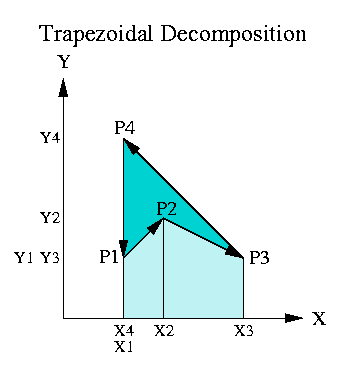University of California, Berkeley EECS Dept, CS Division Jordan Smith SLIDE: Scene Language for Interactive Dynamic Environments Prof. Carlo H. Séquin

 Home Goals Publications People Gallery Assignments Distributions

# Back Face Culling Notes

## Back Face Culling

Back face culling is when you do not render a polygon because its normal is pointing away from the viewer's eye point. A naive way of preforming this check would be to construct a vector from the eye point to a point on the face and compute the dot product of this line of sight vector and the normal vector. If this scalar is positive then the face is pointing away and consequently can be culled. A more sophisticated way of making this check is to transform the face's normal into the projection space, where the line of sight vector is always the negative Z-axis. Then the check for back face culling is just to see if the Z component of the transformed normal is negative.

The problem now is to find a way to transform face normals, and to find a good representation for the normal to make this transformation easy.

## Transforming Planes

If we have a plane vector n = [a, b, c, d] which describes a plane then for any point p = [x, y, z, 1] in that plane the follow equation holds:

nt p = ax + by + cz + d = 0

If for a point p on the plane, we apply an invertible transformation R to get the transformed point p1, then the plane vector n1 of the transformed plane is given by applying a corresponding transformation Q to the original plane vector n where Q is unknown.

p1 = R p
n1 = Q n

We can solve for Q by using the resulting plane equation:

n1t p1 = 0

Begin by substituting for n1 and p1:

(Q n)t (R p) = 0
nt Qt R p = 0

If Qt R = I then nt Qt R p = nt I p = nt p = 0 which is given.

Qt R = I
Qt = R-1
Q = (R-1)t

Substituting Q back into our plane vector transformation equation we get:

n1 = Q n = (R-1)t n

## Transforming Normals

Transforming normals is very similar to transforming planes. A normal norm can be represented by a vector norm = [a, b, c, 0]. This is equivalent to the plane perpendicular the normal and passing through the origin n = [a, b, c, 0]. If we then transform this plane vector by (R-1)t, we will correctly transform the normal for a certain set of transformations:

norm1 = (R-1)t norm

This set of transformations includes our ordinary modelling transformations and the parallel projection transformation where our transformation matrices are always of the form:

 a11 a12 a13 a14 a21 a22 a23 a24 a31 a32 a33 a34 0 0 0 1

For this set of transformations, the d component of n has no effect on the transformed normal direction norm1 because it is multiplied by 0 in the terms for the a1, b1, and c1 components. But our transformations will not stay in this family once we introduce the perspective projection transformation, so we might as well compute and use the full plane equations for our face normals.

So we will represent the normal of face by the best fit plane vector for that face n = [a, b, c, d] where for a point p = [x, y, z, 1] on the face the follow equation holds:

nt p = 0

Now when we apply (R-1) to n:

n1 = (R-1)t n

we will correctly transform the plane and consequently the normal. We are only interested in the orientation of this plane equation, so we can forget the d1 component of n1 to get norm1 = [a1, b1, c1]. norm1 can then be normalized to unit length if necessary.

This is very close to being correct, but unfortunately plane equations have one less degree of freedom than normal vectors. So after the plane vector is transformed the [a1, b1, c1] components can equal either norm1 or -norm1. Fortunately, we can distinguish between these two cases by looking at the determinant of the transformation matrix R.

If Det(R) > 0 then norm1 = [a1, b1, c1]
else norm1 = -[a1, b1, c1]

Det(R) switches sign for every scale transformation with an odd number of negative scale components.

## Computing the Best Fit Plane for a Polygon Loop

We will use Newell's algorithm for computing the normal for a polygon loop which may have noisy data. This algorithm comes close to making a least squares best fit, but computes it in a closed form. This algorithm makes use of the fact that the components of the normal of a polygon are proportional to the signed projected areas of the polygon onto the YZ, XZ, and YZ planes. In the figure below, the white polygon's normal norm = [Area(ProjYZ), Area(ProjXZ), Area(ProjXY)].The areas of the projected polygons can be computed by treating each edge of the projected polygon as a trapezoid with a signed area. In the example below we are looking at the projection onto the XY plane, so the area is proportional to the Z component of the normal. The formula for the area of the trapezoids shown is

Area = width * height / 2 = (xi+1 - xi) (yi + yi+1) / 2

The areas of the trazepoids who are constructed from left pointing polygon edges are considered positive, right pointing polygon edges are considered negative, and vertical edges make zero areas. This follows from the area formula. This choice of sign is consistent with our standard counterclockwise polygon loop orientation. In the figure below, positive trapezoids ([P3, P4]) are colored blue, negative trapezoids ([P1, P2] and [P2, P3]) are colored white, and zero trapezoids ([P4, P1]) do not appear. The net area appears in blue and the areas where positive and negative trapezoids cancel appear in lighter blue.### Formula

With this in mind, if we are given a polygon described by a list of vertices p1, p2, p3, ... pn. We can find a normal vector norm = (nx, ny, nz) whose components are equal to the signed projected areas of the polygon using these equations:

nx = -1
2
 n SUM i = 1
(zi + zi+1) (yi+1 - yi)
ny = -1
2
 n SUM i = 1
(xi + xi+1) (zi+1 - zi)
nz = -1
2
 n SUM i = 1
(yi + yi+1) (xi+1 - xi)

where xn+1 = x1, yn+1 = y1, and zn+1 = z1.

Then we need to find the d value for the complete plane equation n = [a, b, c, d]. We pin down the specific plane by using a pmean which is the average of the vertex values for x, y, and z. We can then find d using norm and pmean:

d = -normt pmean

Now n = [norm, d] = [a, b, c, d] which is the plane equation that best fits the list of vertices of the polygon contour.

## References

• Computer Graphics: Principles and Practice, Foley, vanDam, Feiner, and Hughes, pp. 663-664}
• Computer Graphics: Principles and Practice, Foley, vanDam, Feiner, and Hughes, pp. 216-217}
• Computer Graphics: Principles and Practice, Foley, vanDam, Feiner, and Hughes, pp. 476-477}